Click to Chat

1800-1023-196

+91-120-4616500

CART 0

• 0

MY CART (5)

Use Coupon: CART20 and get 20% off on all online Study Material

ITEM
DETAILS
MRP
DISCOUNT
FINAL PRICE
Total Price: Rs.

There are no items in this cart.
Continue Shopping• Complete JEE Main/Advanced Course and Test Series
• OFFERED PRICE: Rs. 15,900
• View Details

```Revision Notes on Kinematics

Inertial frame of reference:- Reference frame in which Newtonian mechanics holds are called inertial reference frames or inertial frames. Reference frame in which Newtonian mechanics does not hold are called non-inertial reference frames or non-inertial frames.
The average speed vav and average velocity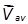of a body during a time interval ?t is defined as,

vav= average speed

= ?s/?t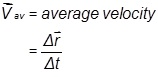Instantaneous speed and velocity are defined at a particular instant and are given byNote:

(a) A change in either speed or direction of motion results in a change in velocity

(b) A particle which completes one revolution, along a circular path, with uniform speed is said to possess zero velocity and non-zero speed.

(c) It is not possible for a particle to possess zero speed with a non-zero velocity.

Average acceleration is defined as the change in velocity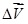over a time interval ?t.The instantaneous acceleration of a particle is the rate at which its velocity is changing at that instant.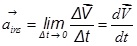The three equations of motion for an object with constant acceleration are given below.

(a) v= u+at

(b) s= ut+1/2 at2

(c) v2=u2+2as

Here u is the initial velocity, v is the final velocity, a is the acceleration , s is the displacement travelled by the body and t is the time.

Note: Take ‘+ve’ sign for a when the body accelerates and takes ‘–ve’ sign when the body decelerates.

The displacement by the body in nth second is given by,

sn= u + a/2 (2n-1)

Position-time (x vs t), velocity-time (v vs t) and acceleration-time (a vs t) graph for motion in one-dimension:

(i) Variation of displacement (x), velocity (v) and acceleration (a) with respect to time for different types of motion.

Displacement(x)

Velocity(v)

Acceleration (a)

(a) At rest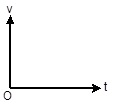(b) Motion with  constant velocity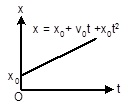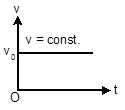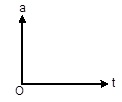(c) Motion with constant acceleration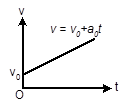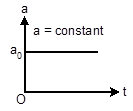(d) Motion with constant deceleration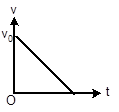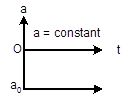Scalar Quantities:- Scalar quantities are those quantities which require only magnitude for their complete specification.(e.g-mass, length, volume, density)

Vector Quantities:- Vector quantities are those quantities which require magnitude as well as direction for their complete specification. (e.g-displacement, velocity, acceleration, force)

Null Vector (Zero Vectors):- It is a vector having zero magnitude and an arbitrary direction.

When a null vector is added or subtracted from a given vector the resultant vector is same as the given vector.

Dot product of a null vector with any arbitrary is always zero. Cross product of a null vector with any other vector is also a null vector.

Collinear vector:- Vectors having a common line of action are called collinear vector. There are two types.

Parallel vector (θ=0°):- Two vectors acting along same direction are called parallel vectors.

Anti parallel vector (θ=180°):-Two vectors which are directed in opposite directions are called anti-parallel vectors.

Co-planar vectors- Vectors situated in one plane, irrespective of their directions, are known as co-planar vectors.

Vector addition is commutative-Vector addition is associative-Vector addition is distributive-Triangles Law of Vector addition:- If two vectors are represented by two sides of a triangle, taken in the same order, then their resultant in represented by the third side of the triangle taken in opposite order.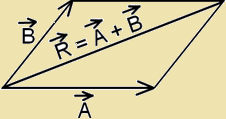Magnitude of resultant vector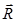:-

R=√(A2+B2+2ABcosθ)

Here θ is the angle between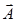and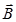.

If β is the angle betweenand,

then,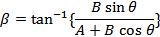If three vectors acting simultaneously on a particle can be represented by the three sides of a triangle taken in the same order, then the particle will remain in equilibrium.

So,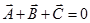R=√(A2+B2+2ABcosθ),Cases 1:- When, θ=0°, then,

R= A+B (maximum), β=0°

Cases 2:- When, θ=180°, then,

R= A-B (minimum), β=0°

Cases 3:- When, θ=90°, then,

R=√(A2+B2), β = tan-1 (B/A)

The process of subtracting one vector from another is equivalent to adding, vectorially, the negative of the vector to be subtracted.

So,Resolution of vector in a plane:-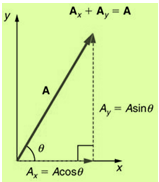Product of two vectors:-

(a) Dot product or scalar product:-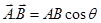,Here A is the magnitude of, B is the magnitude ofand θ  is the angle betweenand.

(i) Perpendicular vector:-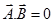(ii) Collinear vector:-

When, Parallel vector (θ=0°),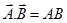When, Anti parallel vector (θ=180°),(b) Cross product or Vector product:-Or,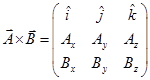Here A is the magnitude of, B is the magnitude of,θ is the angle betweenandand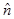is the unit vector in a direction perpendicular to the plane containingand.

(i) Perpendicular vector (θ=90°):-(ii) Collinear vector:-

When, Parallel vector (θ=0°),(null vector)

When, θ=180°,(null vector)

Unit Vector:- Unit vector of any vector is a vector having a unit magnitude, drawn in the direction of the given vector.

In three dimension,Area:-

Area of triangle:-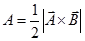Area of parallelogram:-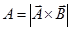Volume of parallelepiped:-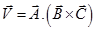Equation of Motion in an Inclined Plane: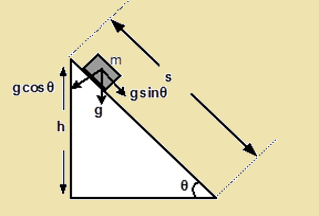(i) Perpendicular vector :-  At the top of the inclined plane (t = 0, u = 0 and a = g sinq  ), the equation of motion will be,

(a) v= (g sinθ)t

(b) s = ½ (g sinθ) t2

(c) v2 = 2(g sinθ)s

(ii) If time taken by the body to reach the bottom is t, then   s = ½ (g sinθ) t2

t = √(2s/g sinθ)

But sinθ =h/s   or s= h/sinθ

So, t =(1/sinθ) √(2h/g)

(iii) The velocity of the body at the bottom

v=g(sinθ)t

=√2gh

The relative velocity of object A with respect to object B is given by

VAB=VA-VB

Here, VB is called reference object velocity.

Variation of mass:- In accordance to Einstein’s mass-variation formula, the relativistic mass of body is defined as,

m= m0/√(1-v2/c2)

Here, m0 is the rest mass of the body, v is the speed of the body and c is the speed of light.

Projectile motion in a plane:- If a particle having initial speed u is projected at an angle θ (angle of projection) with x-axis, then,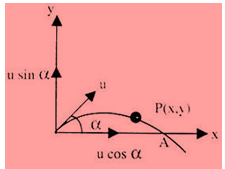Time of Flight, T = (2u sinα)/g

Horizontal Range, R = u2sin2α/g

Maximum Height, H = u2sin2α/2g

Equation of trajectory, y = xtanα-(gx2/2u2cos2α)

Motion of a ball:-

(a) When dropped:-  Time period, t=√(2h/g) and speed, v=√(2gh

(b) When thrown up:- Time period, t=u/g and height, h = u2/2g

Condition of equilibrium:-

(a)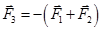(b) |F1+F2|≥|F3|≥| F1-F2|
```### Course Features

• 728 Video Lectures
• Revision Notes
• Previous Year Papers
• Mind Map
• Study Planner
• NCERT Solutions
• Discussion Forum
• Test paper with Video Solution